# AP Calculus AB : Derivatives

## Example Questions

### Example Question #11 : Slope Of A Curve At A Point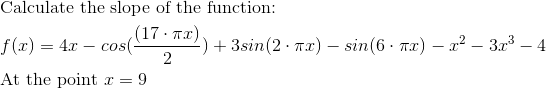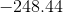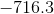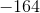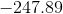Explanation: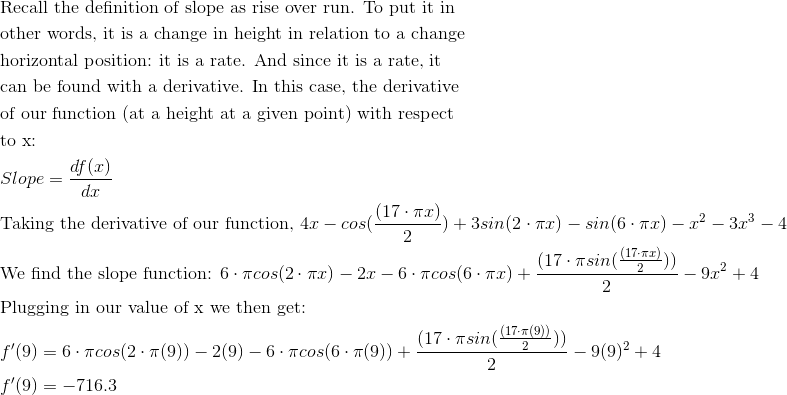### Example Question #34 : Derivative At A Point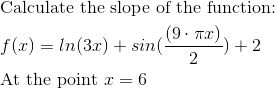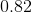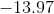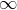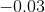Explanation: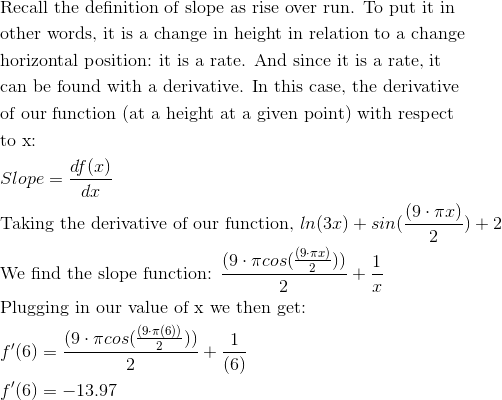### Example Question #35 : Derivative At A Point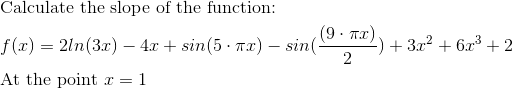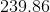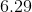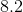Explanation: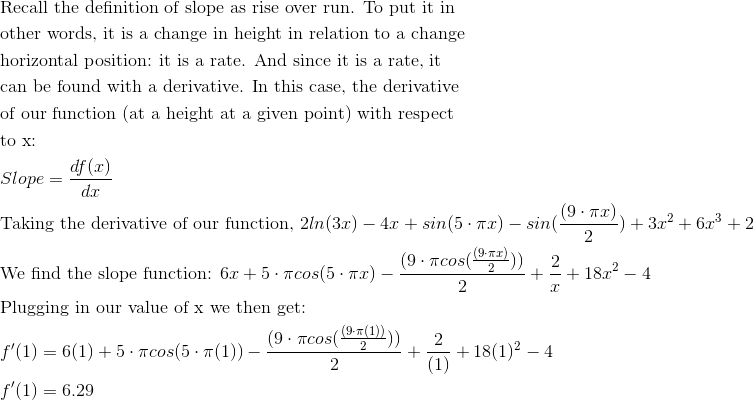### Example Question #131 : Derivatives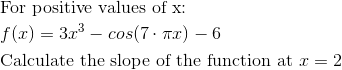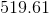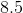Explanation: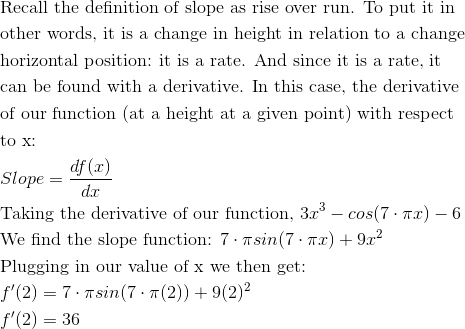### Example Question #131 : Derivatives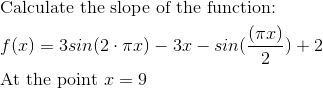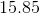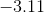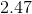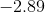Explanation: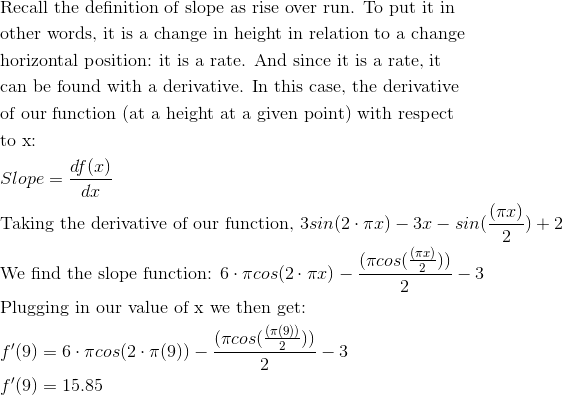### Example Question #31 : Derivative At A Point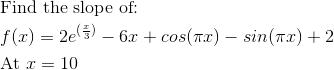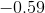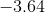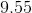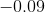Explanation: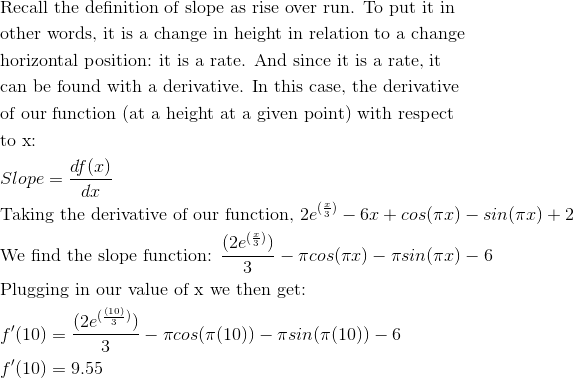### Example Question #23 : Slope Of A Curve At A Point

Find the slope of the line tangent to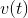when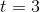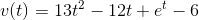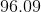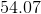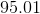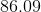Explanation:

Find the slope of the line tangent towhenNow, let's first look at this conceptually. We need to find the slope of a tangent line at a particular value for t. This sounds like a job for a derivative.

We will first find v'(t), then we will plug in three to find v'(3), which will yield our answer.

First, recall:

1)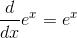2)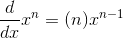These two rules are all that we need to solve this problem: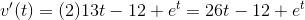So, we have: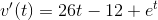Let's plug in 3...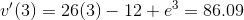### Example Question #132 : Derivatives

Find the slope of the line tangent to the curve of d(t) when t is equal to 0.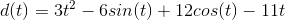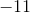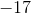Explanation:

Find the slope of the line tangent to the curve of d(t) when t is equal to 0.So, we are asked to find the slope of a tangent line at a particular point. To do so, we need to find the derivative of our function, and then evaluate it at the given value of t.

Let's begin by finding d'(t).

To do so, we need to use the power rule, as well as the rules for sine and cosine.

1) Power Rule2) Sine Rule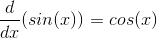3) Cosine Rule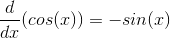Now, we can use all of these to find the derivative of d(t)Becomes: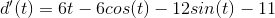Now, we are almost there, but we need to evaluate this derivative when t=0.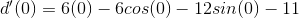This simplifies quite nicely to: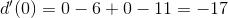So, the slope of our tangent line when t=0 is negative 17.

### Example Question #41 : Derivative At A Point

If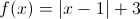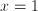, what is the value of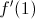?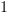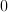Does not exist.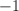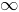Does not exist.

Explanation:

The function is not differentiable at. So the derivative atdoes not exist.

### Example Question #21 : Slope Of A Curve At A Point

Find the slope of the line tangent to the function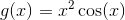at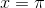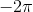Explanation:

The slope of the line tangent to a function is nothing more than the first derivative, which is for this function equal to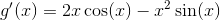found using the following rules: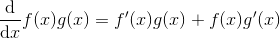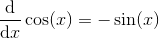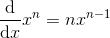Evaluated at the given point, we find the slope of the tangent line equal to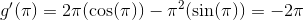### All AP Calculus AB Resources